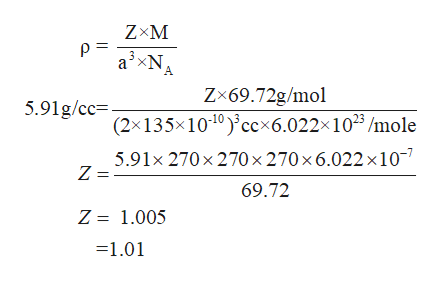# Gallium has a simple cubic unit cell. It has a molar mass of 69.72 g/mol and a density of 5.91 g/cm³. How many atoms are contained within the unit cell?

Question
1 views

Gallium has a simple cubic unit cell. It has a molar mass of 69.72 g/mol and a density of 5.91 g/cm³. How many atoms are contained within the unit cell?

check_circle

Step 1

Given data

Gallium forms a simple cubic cell i.e. value of edge length (a) = 2r

Atomic radii of gallium = 135 x 10-10 cm

Molar mass = 69.72 g/mol

Density = 5.91 g/cc

Step 2

The formula for dens...help_outlineImage TranscriptioncloseZ M p a3xN A Zx69.72g/mo (2x135x1010) ccx6.022x1023/mole 5.91g/cc= 5.91x 270 x 270x 270 x6.022 x 107 Z = 69.72 Z = 1.005 =1.01 fullscreen

### Want to see the full answer?

See Solution

#### Want to see this answer and more?

Solutions are written by subject experts who are available 24/7. Questions are typically answered within 1 hour.*

See Solution
*Response times may vary by subject and question.
Tagged in

### Chemistry